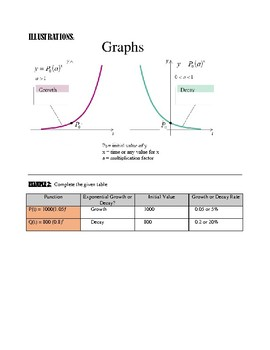EXPONENTIAL GROWTH AND EXPONENTIAL DECAY With ExamplesSubject
Resource Type
Product Rating
3.0
1 Rating
File Type

PDF (Acrobat) Document File

Be sure that you have an application to open this file type before downloading and/or purchasing.

592 KB|9 pages
Share
Product Description
Exponential growth or decay can be modeled using an exponential function of the form:

y= P ( a) ^x

P = initial value of y
x = time or any value for x
a = multiplication factor

i. If a>1 then the function y is an exponential growth where a = 1 + r
and r is the growth rate as a decimal number.

ii. If then the function y is an exponential decay where a = 1 - r
and r is the decay rate as a decimal number.

This document discusses applied problems that relate to exponential growth or decay. The ideas have been laid out so that they are easy to follow. Questions and answers are given. Step by step solutions are also presented.
Total Pages
9 pages
Included
Teaching Duration
1 Week
Report this Resource to TpT
Reported resources will be reviewed by our team. Report this resource to let us know if this resource violates TpT’s content guidelines.